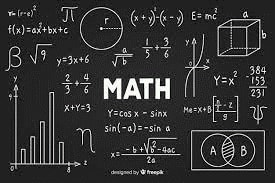# MathSixth Grade Math (2 semesters required)
The goal of sixth grade math is to create a math environment where students will see and understand math as an integral and interesting part of life. The course will review the basic facts of addition, subtraction, division and multiplication of whole numbers and decimals, as well as the addition and subtraction of fractions with unlike denominators. Students will work on graphing and statistics; algebra, basic percent, ratio and probability; perimeter and area; as well as discovering various estimating and problem solving strategies. Students will use a rubric scoring guide and support the six traits of writing through the problem solving process of Mathematical Reasoning and Explanation problems. Students will focus on improving their problem-solving skills as well as their mental math skills.

Seventh Grade Math (2 semesters required)
The seventh grade general math program provides a transition from the basic skills learned in last year to the upper levels of specialized math found in high school programs. Students develop a personal number sense, demonstrate proportional reasoning skills, develop algebraic concepts, extend shape and dimension applications, receive instruction on basic geometry, and develop logical thinking skills. Throughout the course of study, students develop as mathematical problem solvers and learn to communicate mathematically through Mathematical Reasoning and Explanation problems. As students develop a strong foundation, they acquire self-confidence in mathematics, and make connections between mathematics and real life.

Eighth Grade Algebra I (2 semesters required) CADR This is a high school credit class. It is the culmination of middle school mathematics, and prepares students well for successful courses in high school. The fundamental purpose of this course is to formalize and extend the mathematics that students learned in the middle grades. The course focuses on five critical areas: (1) developing fluency writing, interpreting and translating between various forms of linear equations and inequalities and simple exponential functions, and using them to solve problems; (2) comparing and contrasting linear and exponential functions, translating between different representations, using function notation and interpreting arithmetic sequences as linear functions and geometric sequences as exponential functions; (3) using regression techniques to describe linear relationships quantitatively and making judgments about the appropriateness of linear models; (4) extending the laws of exponents to rational exponents, seeing structure in and creating quadratic and exponential expressions, and solving equations, inequalities and systems of equations involving quadratic expressions; and (5) comparing quadratic, linear and exponential functions to model phenomenon. They also identify the real solutions of quadratic equations as the zeroes of a related quadratic function and expand their experience to more specialized functions – absolute value, step and those that are piecewise-defined.

Geometry is not offered at Stella:  It is common for Stella 8th graders to take Geometry online (BYU) in the summer between 8th & 9th grade and then go on to take Algebra 2 in 9th grade. Students who prefer in-person can take geometry in 9th grade.  Some students choose to take both Geometry and Algebra 2 during the same year, concurrently,  in 9th grade. All of these choices must be approved by the student's LWSD High School Counselor, as well as a completed  "Appendix C-Request for Out of District Credit" form before starting.